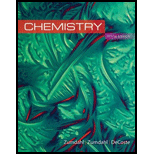# The solubility of Pb(IO 3 )( s ) in a 0.10- M KIO 3 solution is 2.6 × 10 −11 mol/L. Calculate K sp for Pb(IO 3 ) 2 ( s ).### Chemistry

10th Edition
Steven S. Zumdahl + 2 others
Publisher: Cengage Learning
ISBN: 9781305957404

#### Solutions

Chapter
Section### Chemistry

10th Edition
Steven S. Zumdahl + 2 others
Publisher: Cengage Learning
ISBN: 9781305957404
Chapter 16, Problem 48E
Textbook Problem
36 views

## The solubility of Pb(IO3)(s) in a 0.10-M KIO3 solution is 2.6 × 10−11 mol/L. Calculate Ksp for Pb(IO3)2(s).

Interpretation Introduction

Interpretation: The solubility of Pb(IO3)2 and the concentration of KIO3 solution is given. The solubility product of Pb(IO3)2 is to be calculated in a given concentration of KIO3 solution.

Concept introduction: Solubility is defined as the maximum amount of solute that can dissolve at a certain amount of solvent at certain temperature. The solubility product, Ksp is the equilibrium constant that is applied when salt partially dissolve in a solvent. The solubility product of dissociation of AxBy is calculated as,

Ksp=[A]x[B]y

Where,

• x is coefficient of concentration of A .
• y is coefficient of concentration of B .

### Explanation of Solution

Explanation

To determine: The solubility product of Pb(IO3)2 in 0.10M KIO3 .

The solubility product of Pb(IO3)2 in 0.10M KIO3 is 2.6×1013_ .

Given

Solubility of Pb(IO3)2 is 2.6×1011mol/L .

Concentration of KIO3 is 0.10M .

The major species in the solution before dissociation of Pb(IO3)2 are K+,IO3 and H2O . The dissociation reaction of Pb(IO3)2 is,

Pb(IO3)2s)Pb2+(aq)+2IO3(aq)

The ratio of moles between ions is 1:2 .

It is assumed that smol/L of solid is dissolved to reach the equilibrium. The meaning of 1:2 stoichiometry of salt is,

smol/LPb(IO3)21smol/LPb2++2smol/LIO3

The concentrations are as follows,

Initialconcentration(mol/L)Equilibriumconcentration(mol/L)[Pb2+]=0[Pb2+]=s[IO3]=0

### Still sussing out bartleby?

Check out a sample textbook solution.

See a sample solution

#### The Solution to Your Study Problems

Bartleby provides explanations to thousands of textbook problems written by our experts, many with advanced degrees!

Get Started

Find more solutions based on key concepts
What are some characteristics of the adhesive used for Post-it Notes?

General Chemistry - Standalone book (MindTap Course List)

Water-soluble vitamins are mostly absorbed into the lymph. the blood. the extracellular fluid, b and c.

Nutrition: Concepts and Controversies - Standalone book (MindTap Course List)

According to its design specification, the timer circuit delaying the closing of an elevator door is to have a ...

Physics for Scientists and Engineers, Technology Update (No access codes included)